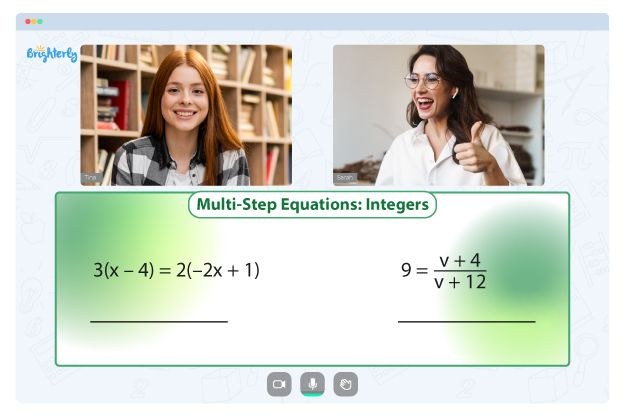# Two Step Equations Worksheets

A two-step equation is an algebraic equation involving two mathematical operations, typically one addition or subtraction operation and one multiplication or division operation. The goal of solving a two-step equation is to isolate the variable, which is the unknown value that needs to be determined.

The first step to solve a two-step equation involves using inverse operations to undo any addition or subtraction operations on the variable. The second step involves using inverse operations to undo any multiplication or division operations on the variable. This results in an equation where the variable is isolated and the equation can be easily solved.

Solving two-step equations is an essential skill for students to master as they progress in their math education. It lays the groundwork for more complex algebraic equations. Kids can use a two step equations worksheets to learn more effectively.

## Benefits of solving two step equations worksheets

### It Encourages Practice

The one and two step equations worksheets is an excellent tool for students to develop their problem-solving skills and reinforce their understanding of two-step equations. In addition, they allow students to practice and apply the concepts they have learned in class.

Students can build their confidence and feel more comfortable solving two-step equations through repeated practice. The more practice problems they solve, the more familiar they become with the process of solving equations, which can help them solve more complex problems easily.

### It Comes with Answer Keys

The two-step equations worksheets has an answer key that allows students to check their work and understand the solution at every step.

The answer key provides a step-wise understanding of how to solve each problem, encouraging students to learn how to solve two-step equations rather than memorize the solution.

### It Improves the Logical and Reasoning Abilities of the Students

Math for Kids

Is Your Child Struggling With Math?
1:1 Online Math TutoringTwo step equations word problems worksheets helps students develop their logical and reasoning abilities. These skills are essential in real-life situations where problem-solving is required, such as budgeting, planning, and decision-making.

By practicing two-step equations on worksheets, students can improve their logical and reasoning skills as they learn to apply mathematical concepts in different contexts. They also develop their critical thinking skills by analyzing problems and determining the most effective solution.

### It Helps to Provide Feedback

Two step equations worksheets pdf can be an excellent tool for teachers to provide feedback to their students. By reviewing the completed worksheets, teachers can easily identify areas where students need improvement and provide targeted guidance on solving problems more effectively.

This feedback mechanism helps students understand their strengths and weaknesses in a particular topic, and they can focus on improving their skills in the areas where they are lacking. By working through the problems at their own pace, students can take the time to understand each concept and identify gaps in their knowledge fully. In addition, feedback allows them to ask questions and seek additional help, ensuring they have a strong foundation in the subject matter.

### It Helps Kids Improve with Difficulty Levels

Worksheets are designed to provide students with a structured and progressive learning experience. The problems in these worksheets are carefully arranged in order of difficulty, which allows students to build their skills and knowledge of the concept gradually. By starting with more straightforward problems and moving on to more complex equations, students can work at their own pace and achieve mastery of the subject matter.

Solving one and two step equations worksheets helps prevent students from becoming overwhelmed and discouraged by complex problems beyond their current level of understanding.

### It Helps Students Understand Real-World Problems

When kids solve two step equations worksheets, they can see the relevance of the concept in everyday situations. This approach helps to engage students and makes learning more enjoyable as kids can relate to the problems they are solving. Real-world examples can also help students develop problem-solving skills that can be applied outside the classroom, which makes the learning experience more meaningful and applicable.

If you are teaching kids in a class and want them to grasp the concept of two step equations quickly enough, download free different two step equation worksheets online. They come in a variety of types and are customized to different learning styles; so, get as many different types as possible and hand them to each kid to see how they learn.

Problems with Equations?• Does your child struggle to grasp equations fundamentals?
• Try studying with an online tutor.

Is your child finding it challenging to master the basics of equations? An online tutor could be the solution.